# Counting Infinity

Infinity is a fascinating idea and it is behind some of the most beautiful results in mathematics. Much of this beauty is accessible to everyone, brought to us by the brilliant Georg Cantor using simple arguments that require little math. I hope to show some of this goodness to people who haven't seen it before. Here we go.

Imagine a shepherd tending a flock of a few dozen sheep. In the morning, the sheep get out of the farm to do sheepish things. In the evening, the shepherd wants to make sure he got all his sheep back. One problem: he can't count. What's a shepherd to do? One solution would be to keep track of the sheep as they go out. For example, he could throw a pebble into a bucket for each sheep leaving the farm. In the evening, a pebble goes out for each returned sheep. At any given time, the number of pebbles in the bucket is exactly equal to that of outstanding sheep. You can picture the sets of sheep and pebbles like so: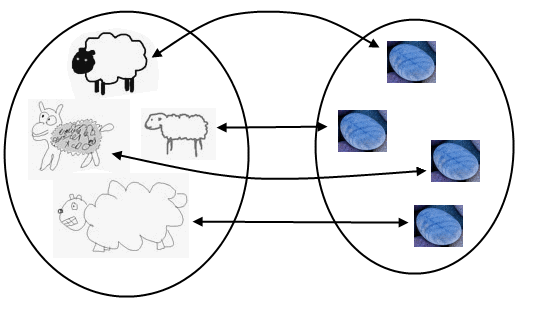Each sheep is paired with a distinct pebble and there are no left overs on either side. In set theory this is a bijection. Even though the sheep have not been counted, we know the sets have the same number of elements. They are equivalent in a way, not because you eat pebbles or throw sheep, though I suppose you could, but because of the bijection. If you accept this premise, which is reasonable enough, then you're in for some fun. Interesting things happen when we use bijections to compare sets nobody can count: the infinite sets. For example, let's compare the natural numbers (1, 2, 3...) to the even natural numbers (2, 4, 6...). It sounds like we should have less even numbers, but lo and behold, we get this: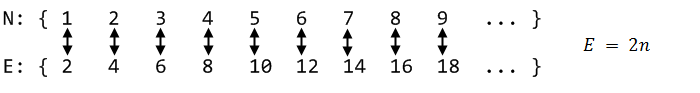Every natural number has been paired with a distinct even number. No left overs. Strangely, these sets are equivalent. It turns out many sets are equivalent to the natural numbers; we call these sets denumerable. For example, the set of Turing Machines is denumerable because there are infinitely many machines and they can each be fully described by a distinct natural number. The integers are yet another example: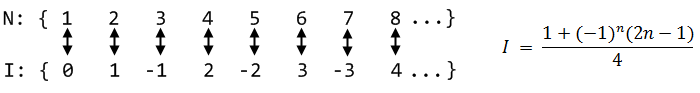Some people see these results as trivial: their intuitive notion is that any "infinity" must be the same. Others see a paradox. How can a part of something be equivalent to the whole? Let's try to give everyone a paradox by finding a set that is not denumerable. How about the rationals? Surely there must be more fractions than natural numbers! Right? Cantor probably thought so, until he discovered a procedure to enumerate the rationals: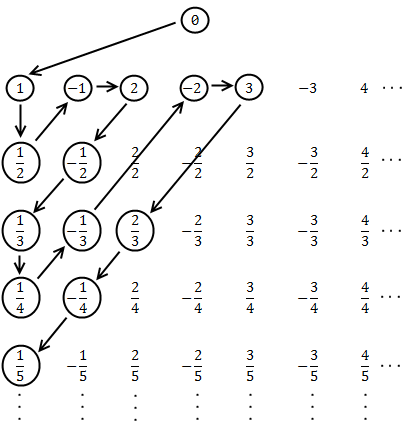Starting at the top and following the pattern, this procedure hits each rational number once. As it moves along, we can associate successive natural numbers with each hit. Here are the first few pairings: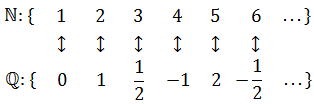It is pretty clear that the arrangement above covers all of the rationals, but the crucial point is that Cantor's pattern will reach any given rational in a finite number of steps. The zigzag is important: if we go off in a single direction (say, across the first row to the right) then we get stuck in the infinitude of that row alone, and there would be rationals left over. The zigzag delivers us from that evil. This argument does not produce an equation for a bijection, but since it builds a listing that contains every rational number, it shows they are denumerable. Conversely, if a set is denumerable its elements can be put into a listing where each element is paired with a natural number.

(As an aside, the 1999 paper Recounting the rationals establishes a precise bijection by using a tree to generate all fractions in reduced form. The tree has all sorts of magical properties, cool stuff. It's an accessible paper, plus Brent Yorgey wrote a great walk through of it.)

All of our sets so far have been denumerable, except for the sheep who were finite. At this point, Cantor might have wondered if maybe there is only one infinity. But then again, if you build a number line using the sets we have seen so far, you end up with holes. The naturals are mere dots on the number line, most fractions fall in a hole: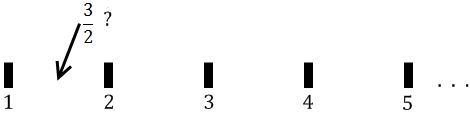The rationals, on the other hand, are dense. Between any two given rationals, there is another rational (an infinite number of them actually). Yet the rational number line also has holes, which are the irrational numbers. Below is a famous irrational number, on whose account its discoverer was allegedly drowned at sea, shown along the rational number line: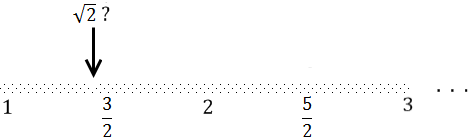Irrational numbers cannot be expressed as a fraction; moreover, when written out as numbers using positional notation (say, as decimal numbers) their digits never settle into any kind of periodic pattern. Fractions, by contrast, eventually settle into a pattern when written out (e.g., 0.333..., 0.25000...). All of the rationals plus all of the irrational numbers make up the real numbers. And those guys form a number line free of holes. Any point in the number line is a real number, any crazy sequence of digits you come up with is a real number. It's the continuum. You might get the impression that irrational numbers are rare, oddballs among the familiar rationals, but that's not the case at all. The sparse number line above is an attempt at visualizing that fact, in addition to showcasing my superior preschool MS Paint skillz. The reals are a vast ocean of irrationals pointed here and there by a fraction, and we'll see why.

So now Cantor pits the real numbers against the naturals. He shows that even a section of the reals - the interval between 0 and 1 - is not denumerable. He uses a proof by contradiction, which is a common way to show that something cannot be true. It goes like this: suppose there is a way to enumerate the reals between 0 and 1. Then there is a listing containing every real number in that interval, each one associated with a different natural number. It might look like this: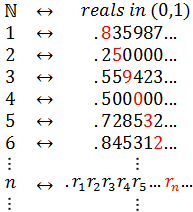The actual numbers shown above are not important, since the complete list is infinite and has every real number in the interval. Some of the reals up there are clearly rationals and have settled into a periodic pattern (0.250... and 0.500...). The others look pretty random, they could be irrational or maybe they haven't settled into their periodic patterns yet. Either way, for each number the digits go on indefinitely, rationals in a pattern (possibly of zeros) and irrationals randomly.

Notice how the numbers in red form a diagonal, which is itself infinite. Let's build a number using that diagonal. Going down the list, for each row we pick a digit that is different than the digit in red. For example, we could add 1 to each digit (9 becomes 0, no carry). You can use any rule as long as the digit is different. Here are the first few digits we get by adding 1 to the red diagonal digits: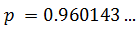The digits of this number p are random and go on infinitely; it is a real number. But due to how we have defined p, it is different from every other number in the list. It differs in at least one digit. Even though the list is infinite, this number is not in it! But then, this list was supposed to have all real numbers so we have a contradiction. Hence our initial assumption must be false; the reals are not denumerable.

The notion of different infinities was a revolutionary one in mathematics. It is a stunning result which ignited the mother of all mathematician flame wars. This was actually the second proof Cantor offered for the non-denumerability of the continuum. The first proof is also valid, but slightly more technical. Cantor's diagonal argument however is amazingly simple and highly original, as brilliant as its conclusion. It went on to feature prominently in other intellectual landmarks. Alan Turing used it while proving that a Turing Machine cannot predict the behavior of other machines (specifically, predict whether another machine is "cycle-free", which is now known as the Halting Problem). Gödel used it in his Incompleteness Theorem discussed in Gödel, Escher, Bach. Diagonalization is crucial in recursion theory and complexity theory.

In order to capture these new concepts, Cantor proposed cardinality as a measure of how numerous a set is. A finite set's cardinality is simply how many elements it has, say 49 for our set of sheep. But the cardinality of an infinite set is expressed by a transfinite number. To make them more esoteric, Cantor used the Hebrew letter aleph for these numbers. By definition, the naturals took aleph zero,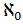, for their cardinality. Every denumerable set has cardinality. The cardinality of the reals was defined as c, and Cantor spent years trying to discover whether c =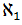, that is, whether the cardinality of the reals is the 'next bigger one' after the naturals. So that explains the name in Mona Lisa Overdrive and also the handle for Aleph One, who wrote the classic paper on stack buffer overflows during the Phrack renaissance.

This concludes our first foray into the infinities. There's way too much to talk about. If there's interest I'll write a follow up to see where else infinity leads us. Iterative rather than upfront blogging :) Meanwhile, Journey Through Genius is a great book that does for many theorems, including Cantor's, what I did on this post. Only much better. Thanks for reading!